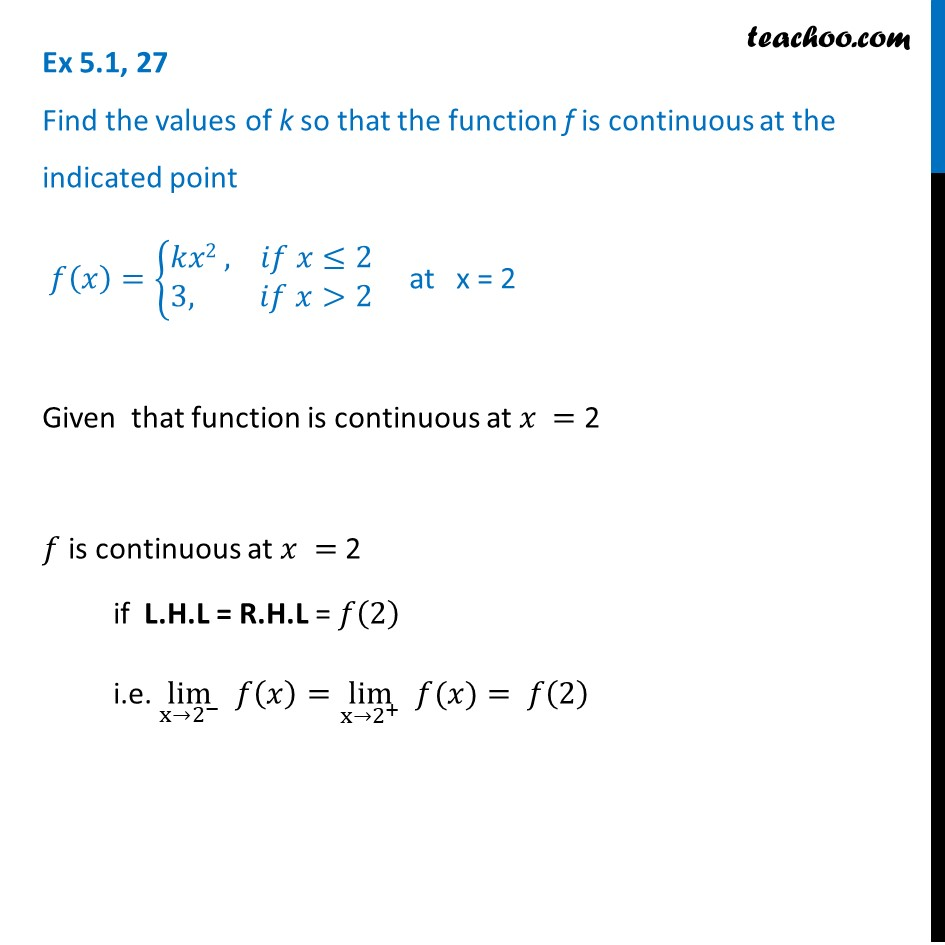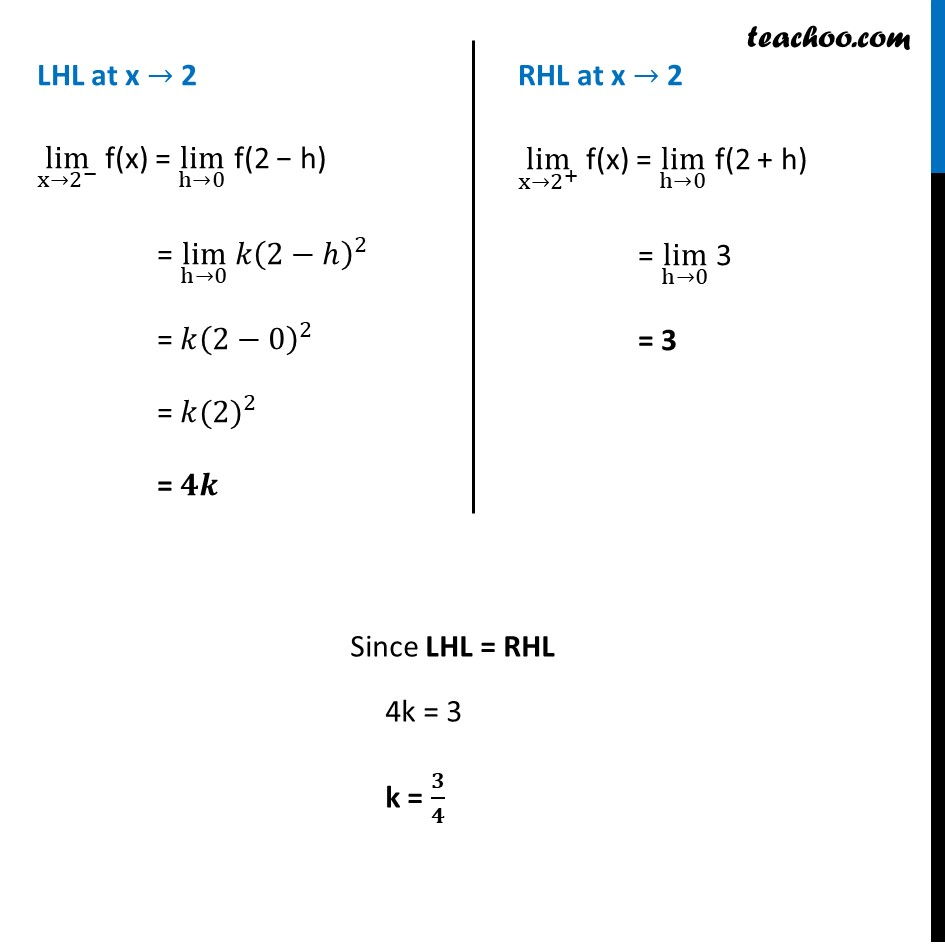1. Chapter 5 Class 12 Continuity and Differentiability (Term 1)
2. Serial order wise
3. Ex 5.1

Transcript

Ex 5.1, 27 Find the values of k so that the function f is continuous at the indicated point 𝑓(𝑥)={█(𝑘𝑥2 , 𝑖𝑓 𝑥≤2@3, 𝑖𝑓 𝑥>2)┤ at x = 2Given that function is continuous at 𝑥 = 2 𝑓 is continuous at 𝑥 = 2 if L.H.L = R.H.L = 𝑓(2) i.e. lim┬(x→2^− ) 𝑓(𝑥)=lim┬(x→2^+ ) " " 𝑓(𝑥)= 𝑓(2) LHL at x → 2 lim┬(x→2^− ) f(x) = lim┬(h→0) f(2 − h) = lim┬(h→0) 〖𝑘(2−ℎ)〗^2 = 〖𝑘(2−0)〗^2 = 〖𝑘(2)〗^2 = 𝟒𝒌 RHL at x → 2 lim┬(x→2^+ ) f(x) = lim┬(h→0) f(2 + h) = lim┬(h→0) 3 = 3 RHL at x → 2 lim┬(x→2^+ ) f(x) = lim┬(h→0) f(2 + h) = lim┬(h→0) 3 = 3 RHL at x → 2 lim┬(x→2^+ ) f(x) = lim┬(h→0) f(2 + h) = lim┬(h→0) 3 = 3 RHL at x → 2 lim┬(x→2^+ ) f(x) = lim┬(h→0) f(2 + h) = lim┬(h→0) 3 = 3 Since LHL = RHL 4k = 3 k = 𝟑/𝟒

Ex 5.1